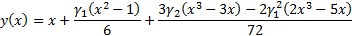/

### Derivation of the Cornish-Fisher asymptotic expansion

A common methodology within risk management circles for estimating the shape of a fat-tailed return distribution is to make use of the Cornish-Fisher asymptotic expansion, see e.g. Abramowitz and Stegun (1970). The Cornish Fisher asymptotic expansion in effect takes into account non-Normality, and thus by implication moments higher than the second moment, by using a formula in which terms in higher order moments explicitly appear. Most commonly the focus is on the fourth-moment version of this expansion, since it merely uses moments up to and including kurtosis. In effect, the fourth-moment Cornish Fisher approach aims to provide a reliable estimate of the distribution’s entire quantile-quantile plot merely from the first four moments of the distribution, i.e. its mean, standard deviation, skew and kurtosis.

The approach works as follows. Let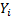be identically distributed random variables. Let the cumulative distribution function of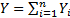be denoted by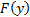. Then the (Cornish-Fisher) asymptotic expansion (with respect to) for the value of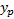such that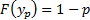is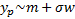where: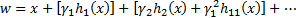Here terms in brackets are terms of the same order with respect to,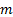is the mean of the distribution,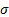the standard deviation of the distribution and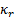are the distribution’s cumulants, i.e. the coefficients of the following power series expansion for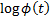where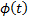is the distribution’s characteristic function.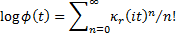The cumulants are related to the moments of the distribution via the relationship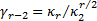(for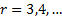) where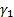is the skew(ness),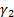is the (excess) kurtosis etc.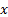is the relevant cumulative Normal distribution point, i.e. the value for which: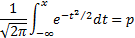and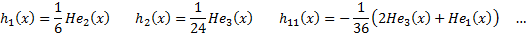The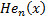are the Hermite polynomials, i.e.: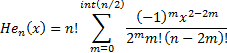In effect, if we are using a fourth moment Cornish-Fisher adjustment then this means estimating the shape of a quantile-quantile plot by the following cubic, whereis the skew andis the kurtosis of the distribution: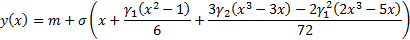For standardised returns (with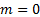and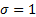), this simplifies to: